﻿ 基于以太坊智能合约的密封式区块链拍卖可信方案的研究

# 基于以太坊智能合约的密封式区块链拍卖可信方案的研究Research on Trusted Scheme of Sealed Blockchain Auction Based on Ethereum Smart Contract

Abstract: In order to solve the problems of traditional auction methods such as low transparency and high costs and enhance the transparency and credibility of auctions, this paper proposes a sealed auction scheme based on blockchain. Firstly, the goods shall be put on blockchain for bidding and price un-covering; then in order to ensure the security of the transaction funds, the funds trusteeship after the auction is designed in this scheme. According to the logic of multi-signature, the final fund flow will be decided by voting. Meanwhile, this scheme optimizes the auction process by using Keccak256 encryption algorithm, elliptic curve signature algorithm ECDSA and RSA digital signature algorithm by stages. Finally, through the security analysis of the credibility of this scheme, it is proved that the scheme is secure under the condition that smart contracts are not forged.

1. 引言

2. 基于区块链的密封拍卖的方案设计

2.1. 方案介绍以及系统平台的选择

2.2. 密封出价的解决方案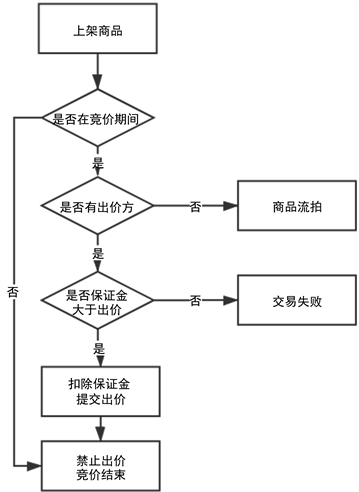Figure 1. Sealed bid flowchart

2.3. 揭价公示的解决方案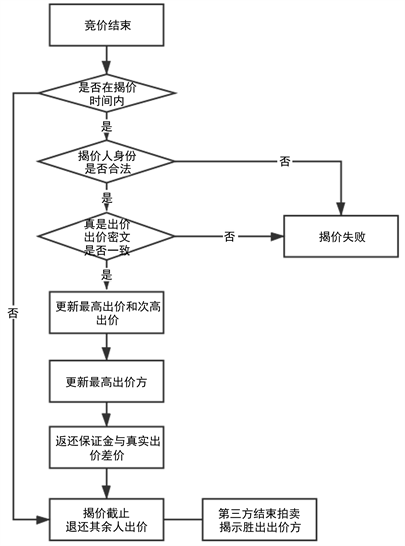Figure 2. Price uncovering flowchart

2.4. 资金托管的解决方案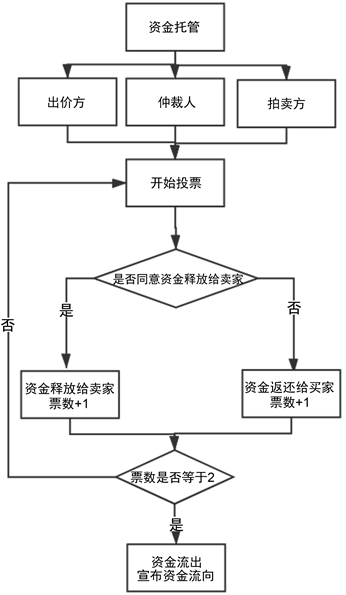Figure 3. Funds trusteeship flow chart

3. 方案主要算法的设计及智能合约的交互细节

3.1. 密封出价方案中keccak算法的设计

keccak256算法是SHA3标准下提出的一种可扩展输出函数SHAKE128的核心算法，而完整的keccak算法描述比较复杂，由于篇幅有限，本文只介绍keccak256加密算法的应用与实现。

keccak算法  采用了海绵结构，基本公式为：

$b=r+c$

$z=SPONGE\left[f\left(b\right),pad,r\right]\left(M,l\right)$ (1)

$M=amount+secretText$

$keccak\left[c\right]\left(M,l\right)=SPONGE\left[f\left(b\right),pad\left(10\ast 1\right),r\right]\left(M,l\right)$

$sealedBid=keccak\left[c\right]\left(M,l\right).toString\left(hex\right)$ (2)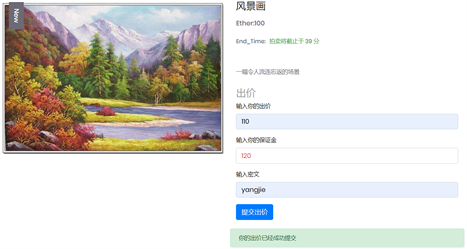Figure 4. Affect picture of sealed bidFigure 5. Affect picture of price uncovering

3.2. 交易数据的确认——数字签名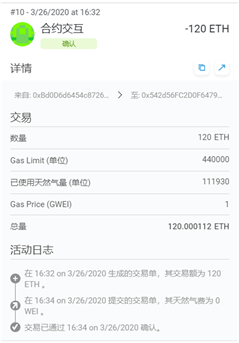Figure 6. Fund transaction data chart

3.2.1. 椭圆曲线数字签名算法ECDSA应用与优势分析

1) 签名流程：

2) 验签流程：

3.2.2. 资金托管中多重签名逻辑的改进与优化

$n=pq$，其中p、q为素数，设 $p=q={z}_{n}$，且定义 $K=\left\{\left(n,p,q,e,d\right):ed=1\mathrm{mod}\phi \left(n\right)\right\}$

$si{g}_{k}\left(x\right)={x}^{d}\mathrm{mod}n$

$ve{r}_{k}\left(x,y\right)=true\text{\hspace{0.17em}}OR\text{\hspace{0.17em}}false$ (3)

${d}_{k}\left({e}_{k}\left(y\right)\right)={x}^{ed}=x\mathrm{mod}n$ (4)

${M}_{2}=HASH\left(a\right)$ (4)

${M}_{3}=HASH\left(b\right)$ (5)

${M}^{\prime }=bytes\left(M+T\right)$

$si{g}_{k}\left({M}^{\prime }\right)={{M}^{\prime }}^{d}\mathrm{mod}n$

$ve{r}_{k}\left({M}^{\prime }\right)=true\text{\hspace{0.17em}}OR\text{\hspace{0.17em}}false$ (7)

${M}^{\prime }$ 进行签名，然后再传给合约使用用户公钥解密后，将拆分开成M和T两部分，根据M判断用户的选择，根据T判断用户签名时间的合法性。相比第一种方案，减少了资金流转过程中过多的运算提高了效率，同时依赖时间戳在一定程度上可以防重放攻击，加强了交易的安全性。本文基于第二种方案投票的多签效果如图7Figure 7. Affect picture of voting in funds trusteeship

3.3. 智能合约交互的业务细节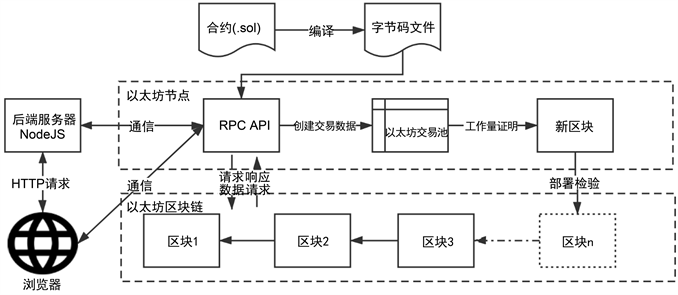Figure 8. Business interaction diagram of auction scheme

3.4. 智能合约交互的技术细节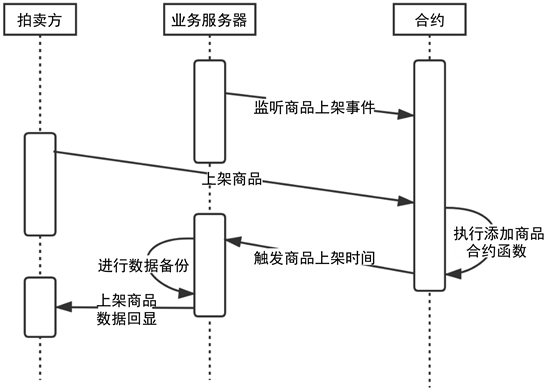Figure 9. The logic of contract interaction about putting goods for bidding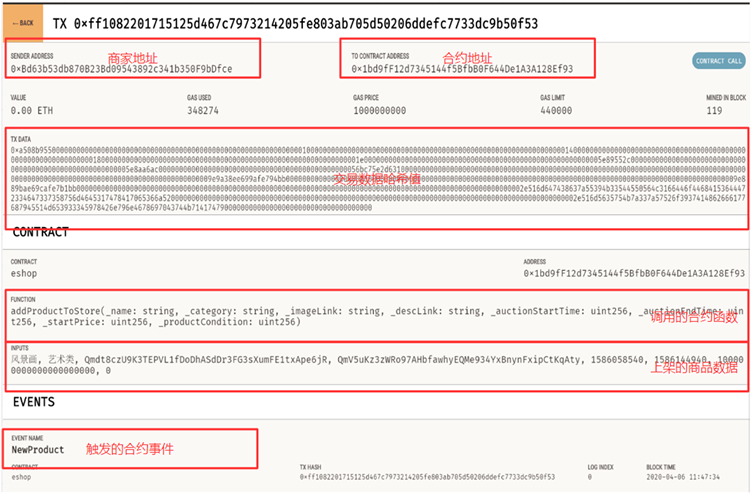Figure 10. Details of transaction block

4. 安全性分析

4.1. 交易数据的安全性分析

4.2. 算法的安全性分析

4.2.1. keccak256算法的安全性分析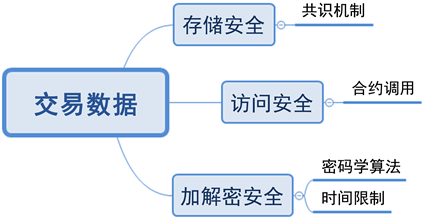Figure 11. Safety summary of transaction data about sealed auction based on blockchain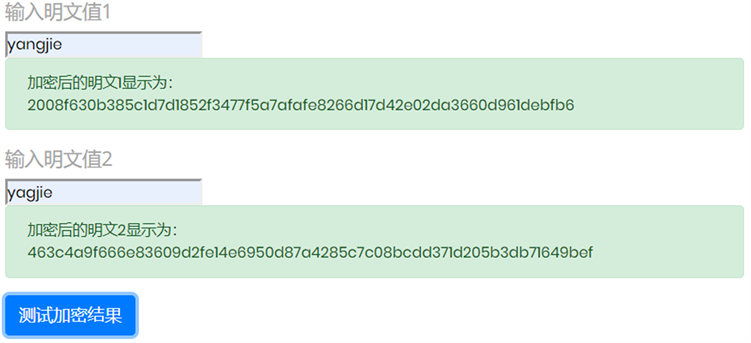Figure 12. The comparison of keccak256 encryption

4.2.2. RSA签名算法的安全性分析

1) 群组内的成员，即使不知道 的分解，仍然可以解密其他人的消息。

$\left\{\begin{array}{l}{e}_{1}{d}_{1}\equiv 1\mathrm{mod}\phi \left(n\right)\\ {e}_{2}{d}_{2}\equiv 1\mathrm{mod}\phi \left( n \right)\end{array}$

${e}_{1}{{d}^{\prime }}_{1}\equiv 1\mathrm{mod}\left({e}_{2}{d}_{2}-1\right)$ (8)

2) 群组外人员截获到发送给群组中不同人的同一消息，而这两个加密指数是互素的，则明文可以不用任何一个解密密钥来恢复。

$\left\{\begin{array}{l}{c}_{1}\equiv {m}^{{e}_{1}}\mathrm{mod}n\\ {c}_{2}\equiv {m}^{{e}_{2}}\mathrm{mod}n\end{array}$

${c}_{1}^{r}{c}_{2}^{s}\equiv {m}^{r{e}_{1}+s{e}_{2}}\mathrm{mod}n\equiv m\mathrm{mod}n$ (9)Table 1. The prevention methods of RSA signature algorithm

4.2.3. ECDSA签名算法的安全性分析

5. 总结

NOTES

*通讯作者。

 Galal, H.S. and Youssef, A.M. (2018) Verifiable Sealed-Bid Auction on the Ethereum Blockchain. In: Proceedings of International Conference on Financial Cryptography and Data Security, Springer, Berlin, Heidelberg, 265-278.
https://doi.org/10.1007/978-3-662-58820-8_18

 李玮. C2C电子商务模式的信任修复机制与在线冲突解决的研究[D]: [硕士学位论文]. 北京: 北京交通大学, 2014.

 张帅. 一种密封式电子拍卖方案的构造[D]: [硕士学位论文]. 重庆: 西南大学, 2019.

 刘峰. 区块链热与企业机遇[J]. 企业管理, 2018(6): 19-21.

 Vickrey, W. (1961) Counterspeculation, Auctions, and Competitive Sealed Tenders. The Journal of Fi-nance, 16, 8-37.

 Ethereum White Paper. A Next-Generation Smart Contract and Decentralized Application Platform.
https://github.com/ethereum/wiki/wiki/White-Paper

 Kiayias, A. and Panagiotakos, G. (2016) Speed-Security Tradeoff s in Blockchain Protocols.

 Bertoni, G., Daemen, J., Peeters, M., et al. (2009) Keccak Sponge Function Family Main Document. Submission to NIST (Round 2), 3, 320-337.

 Diffie, W. and Hellman, M. (1976) New Directions in Cryptography. IEEE Transactions on Information Theory, 22, 644-654.
https://doi.org/10.1109/TIT.1976.1055638

 Wikipedia (2020) Elliptic Curve Digital Signature Algorithm.
https://en.wikipedia.org/wiki/Elliptic_Curve_Digital_Signature_Algorithm

 Wood, G. (2014) Ethereum Yellow Paper.
https://github.com/ethereum/yellowpaper

 张岩, 张爱丽. 数字签名算法RSA与ECDSA的比较与分析[J]. 科协论坛(下半月), 2010(2): 102-103.

 松本晋一, 穴田啓晃, 川本純平, 等. 次世代暗号通貨プラットフォームEthereumの実験的評価[J]. コンピュータセキュリティシンポジウム 2015 論文集, 2015(3): 1151-1158.

 曹迪迪, 陈伟. 基于智能合约的以太坊可信存证机制[J]. 计算机应用, 2019, 39(4): 1073-1080.

 Wikipedia, 雪崩效应.
https://zh.wikipedia.org/wiki/雪崩效应, 2019.

 高磊. 基于RSA公钥算法的数字签名技术研究[D]: [硕士学位论文]. 绵阳: 西南科技大学, 2015.

 Kleinjung, T., Aoki, K., Franke, J., et al. (2010) Factorization of a 768-Bit RSA Modulus. In: Proceedings of Annual Cryptology Conference, Springer, Berlin, Heidelberg, 333-350.
https://doi.org/10.1007/978-3-642-14623-7_18

 Pollard, J.M. (1974) Theorems on Factorization and Primality Testing. Mathematical Proceedings of the Cambridge Philosophical Society, 76, 521-528.
https://doi.org/10.1017/S0305004100049252

 Wiener, M.J. (1990) Cryptanalysis of Short RSA Se-cret Exponents. IEEE Transactions on Information Theory, 36, 553-558.
https://doi.org/10.1109/18.54902

 王起月. 基于椭圆曲线的数字签名算法研究[D]: [硕士学位论文]. 洛阳: 河南科技大学, 2018.

 何倩. 基于全同态加密的电子投票方案研究[D]: [硕士学位论文]. 杭州: 浙江理工大学, 2018.

 余瑞成. 基于安全比较协议的区块链密封式拍卖方案研究[D]: [硕士学位论文]. 咸阳: 西北农林科技大学, 2019.

Top